• ## RLC串联电路

千次阅读 2018-09-23 11:10:06
RLC串联电路，电压Us维持不变，若将Xc=10欧姆，若将L短路，电路的电流有效值与原电路的一样，则求Xl？
RLC串联电路，电压Us维持不变，若将Xc=10欧姆，若将L短路，电路的电流有效值与原电路的一样，则求Xl？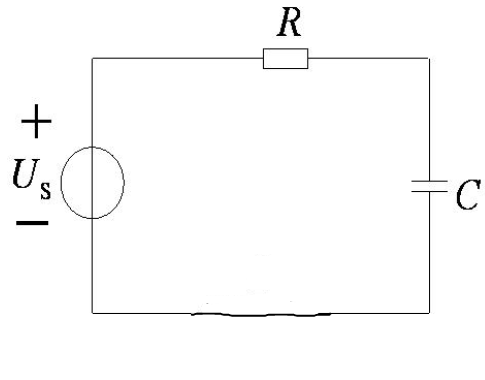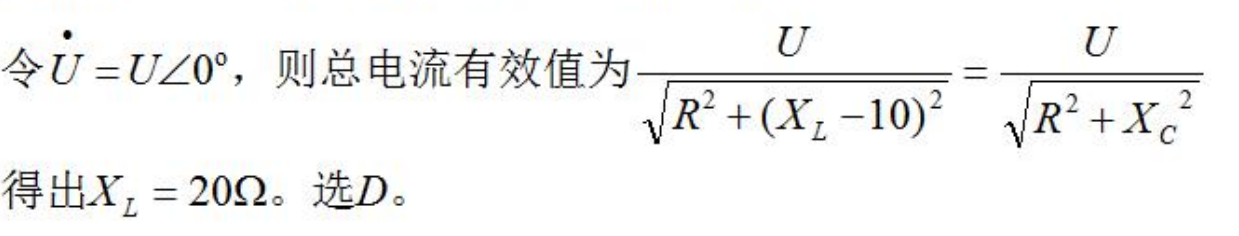展开全文• ## RLC串联电路

千次阅读 2019-06-12 18:24:55
阻抗串联和并联 电路中的谐振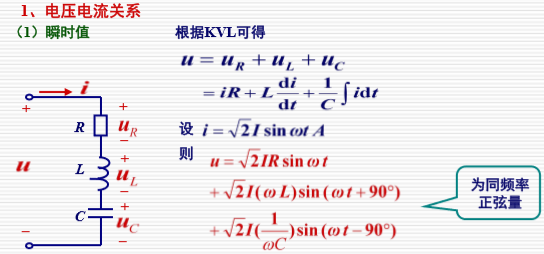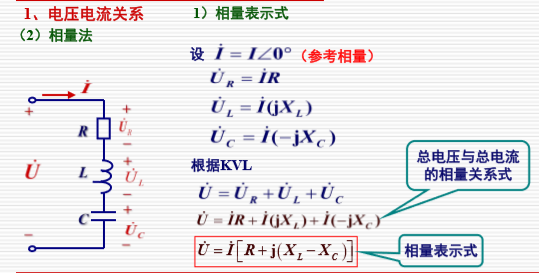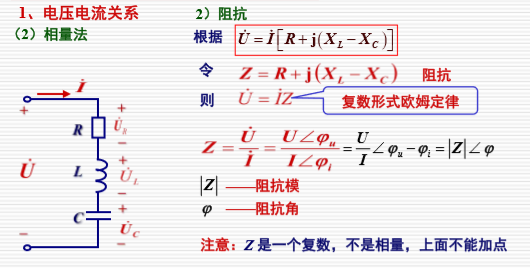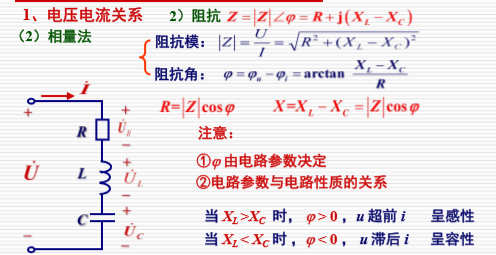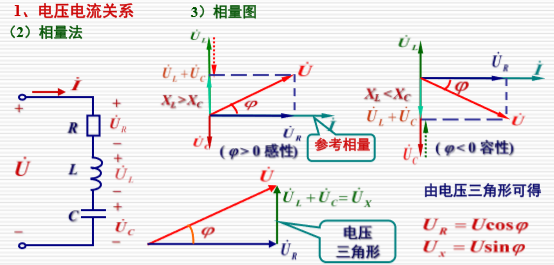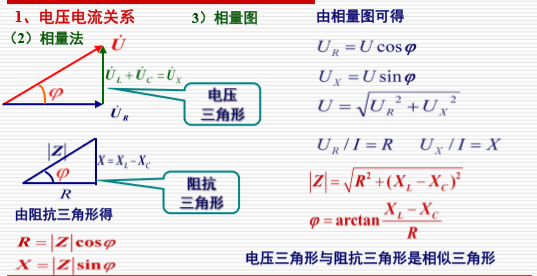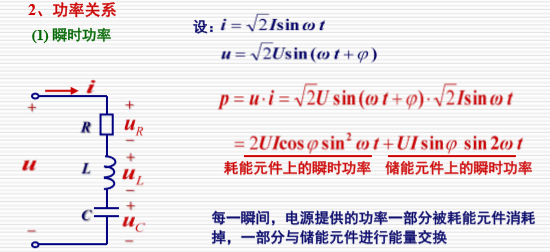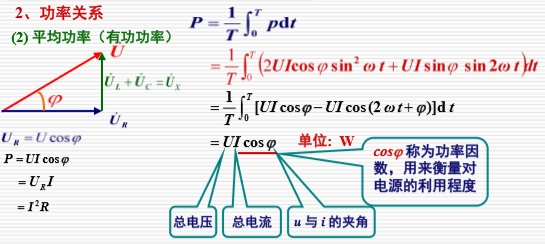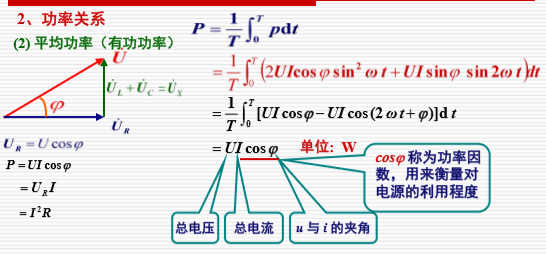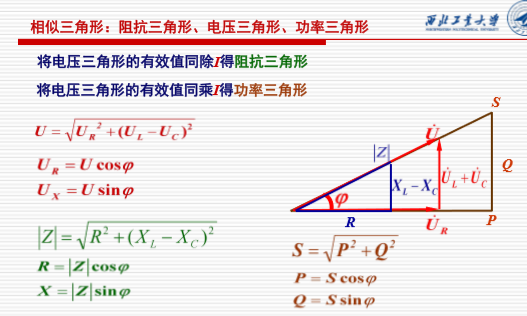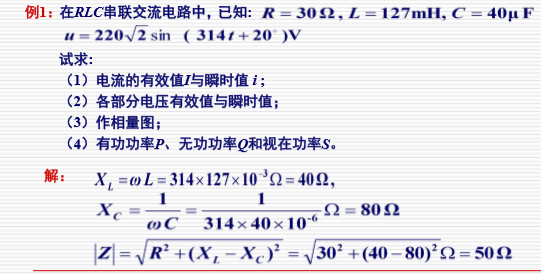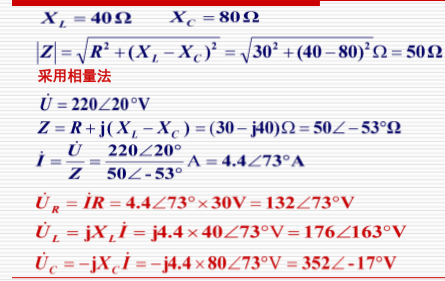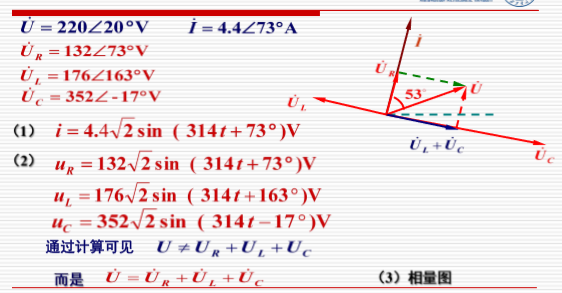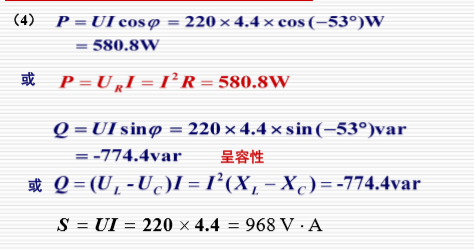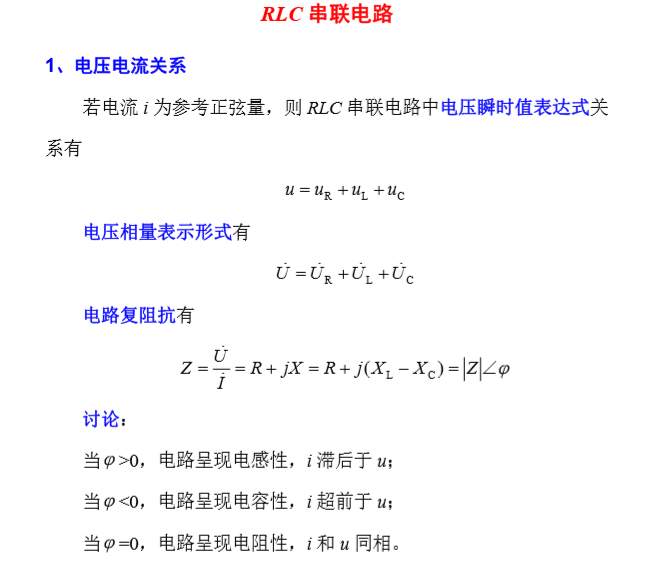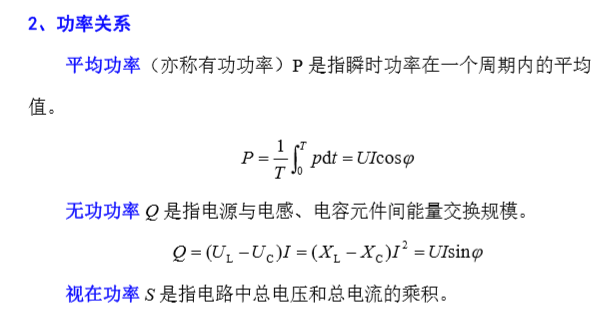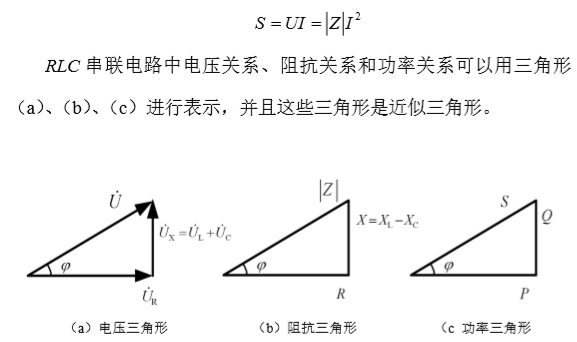阻抗串联和并联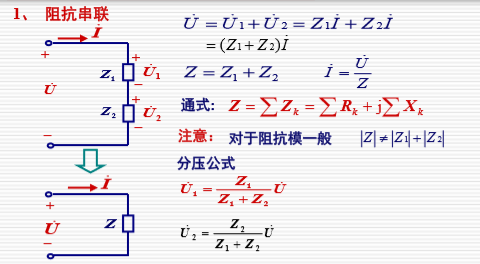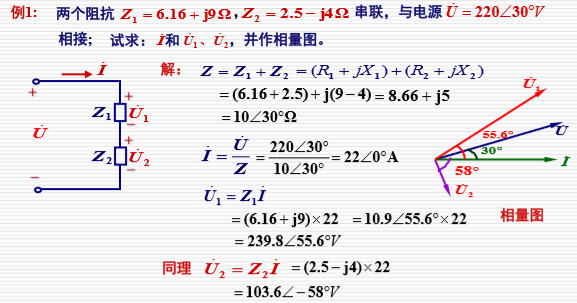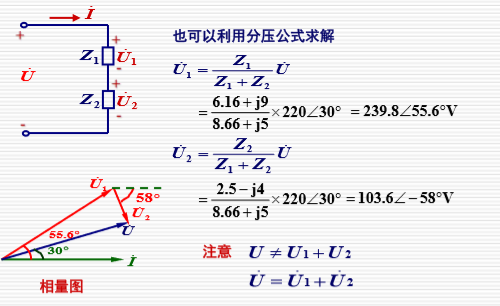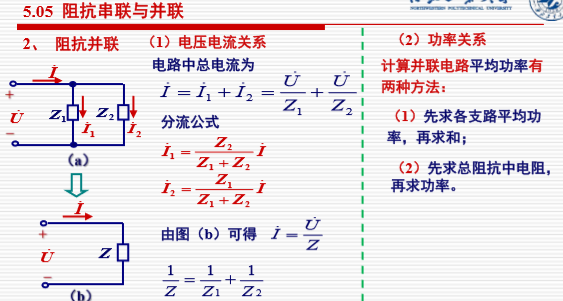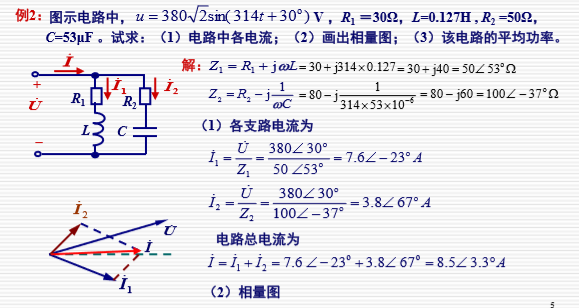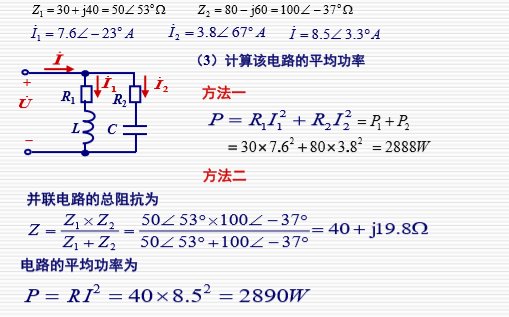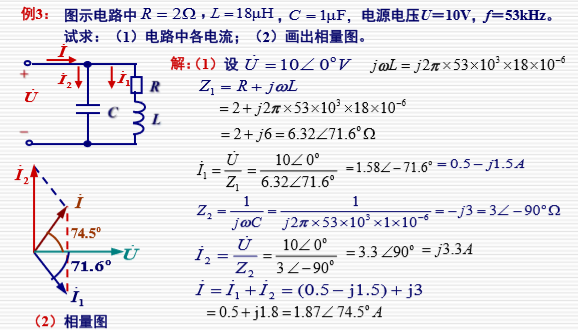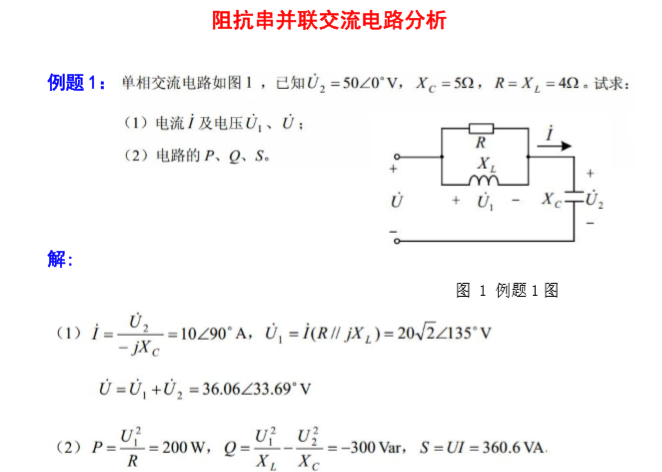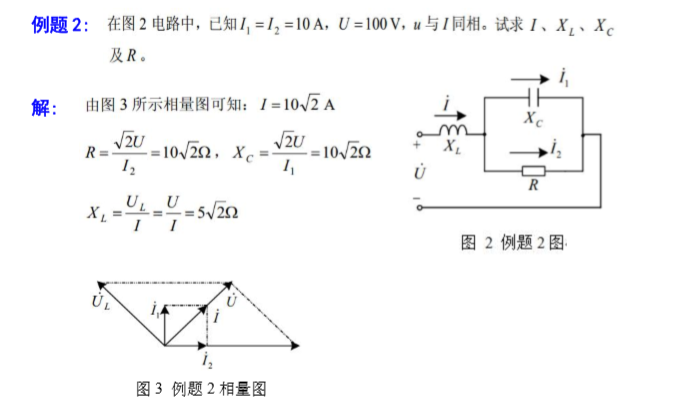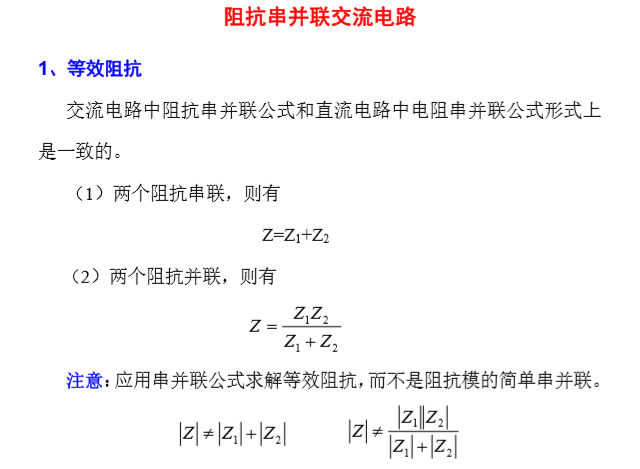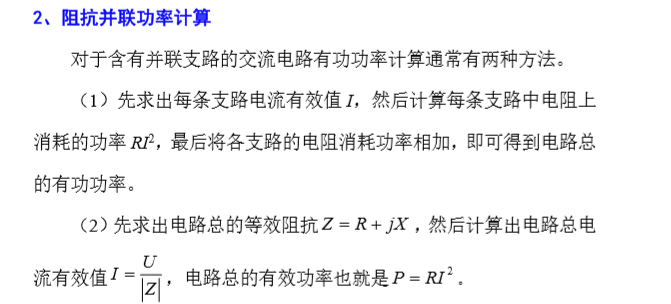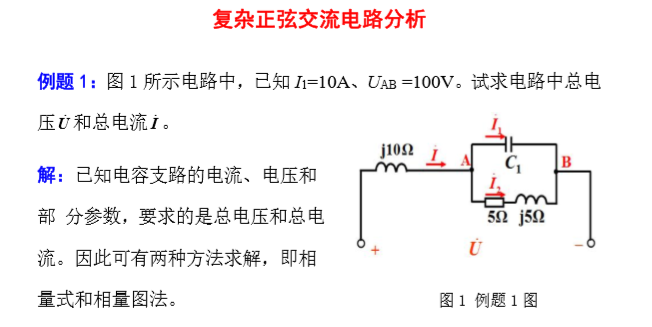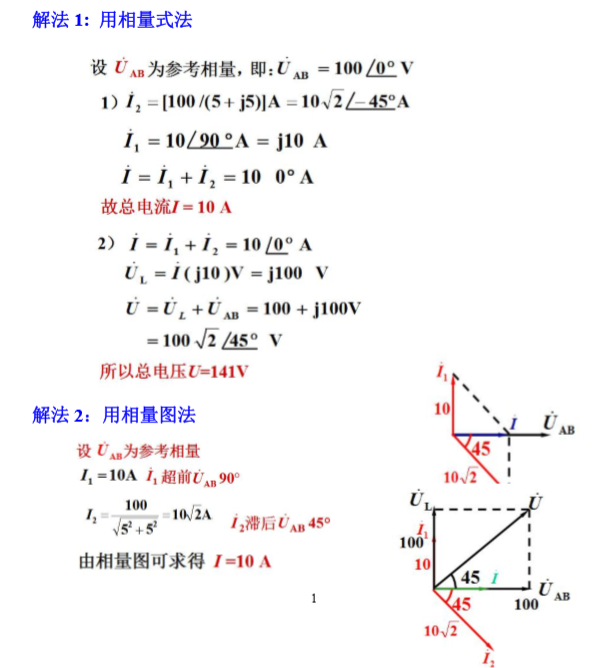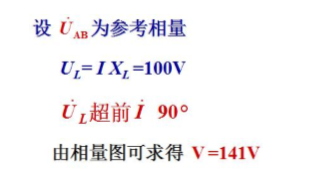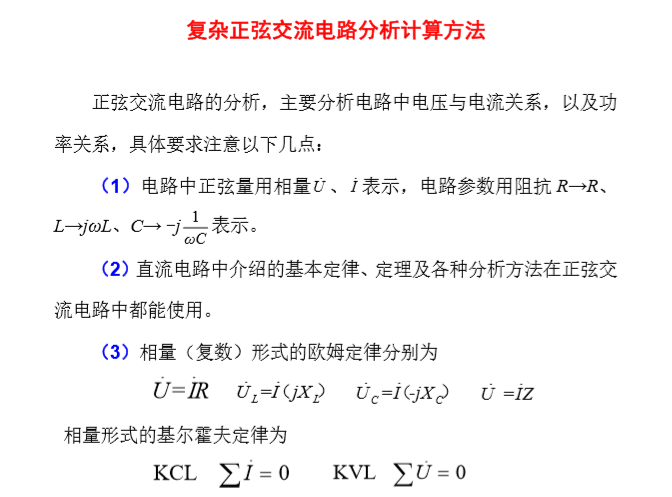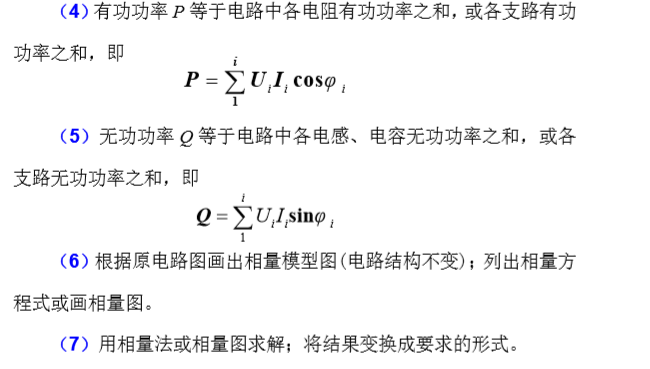电路中的谐振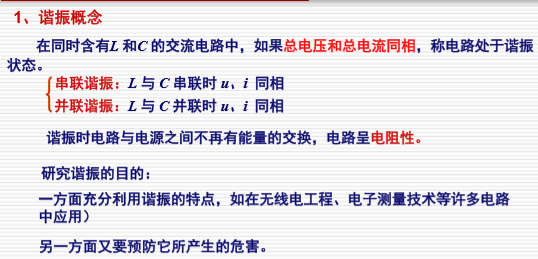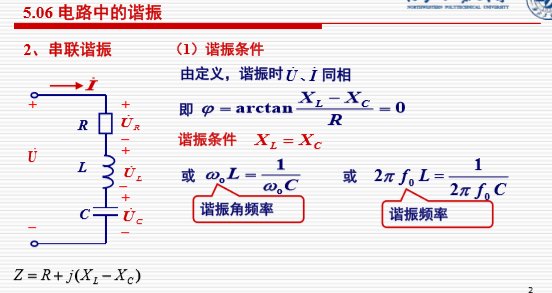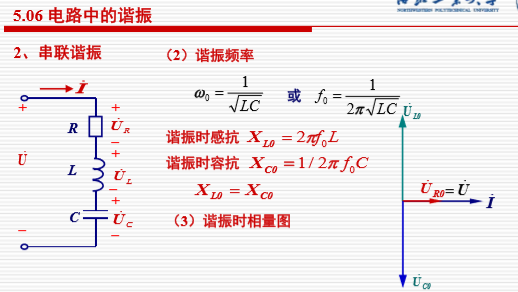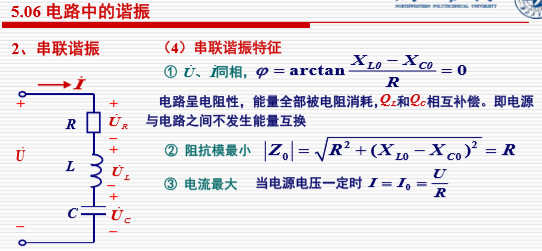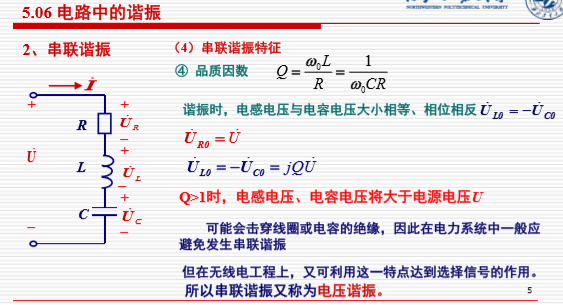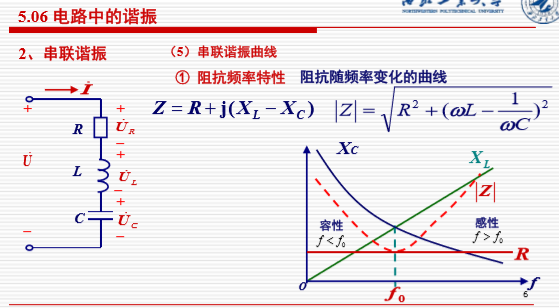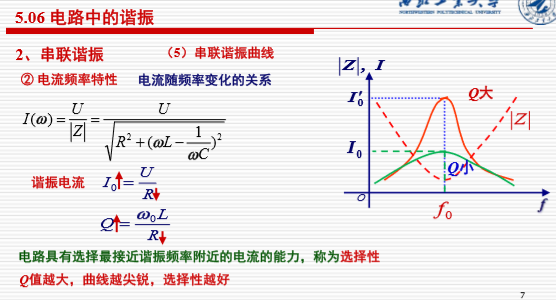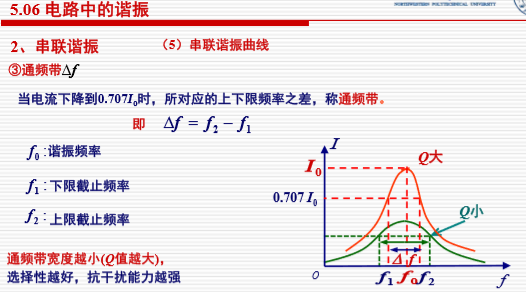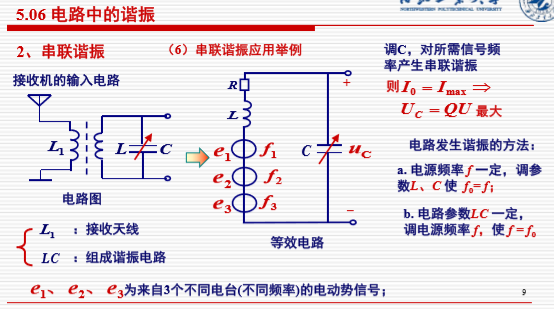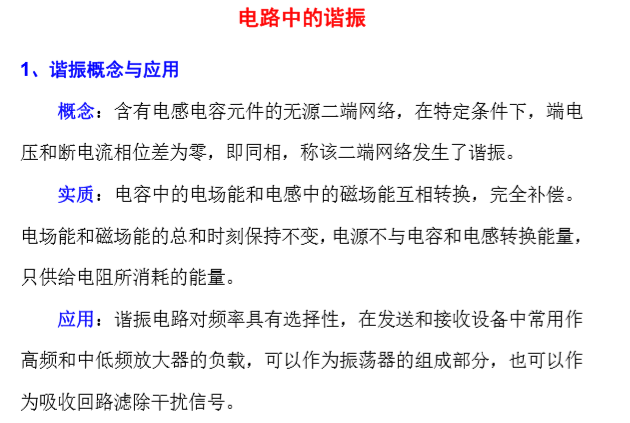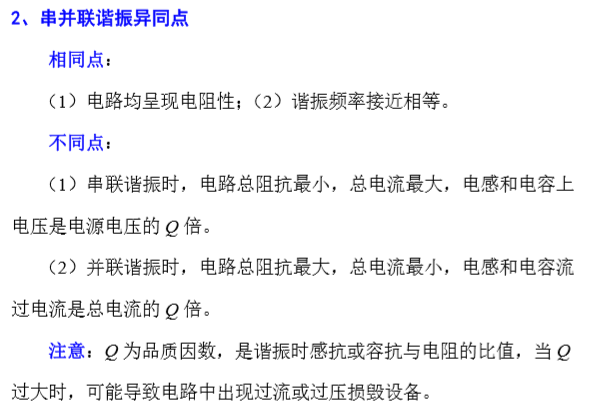展开全文• 摘要：基于探索RLC串联电路谐振特性仿真实验技术的目的，采用Multisim10仿真软件对RLC串联电路谐振特性进行了仿真实验测试，给出了几种Multisim仿真实验方案，介绍了谐振频率、上限频率、下限频率及品质因数的测试和...
• rlc串联电路谐振介绍 谐振电路的阻抗、电压于电流以及它们之间的相位差、电路与外界的能力交换等均处于某种特殊状态，同时具有电感、电容两类元件的电路在一定条件下会发生谐振现象。因而在实际中有重要的应用，如在...
• 分析了非线性RLC串联电路的电路方程和参数，并利用梅利尼科夫（Melnikov）方法推断出非线性RLC串联电路中存在的混沌现象。最后通过计算机模拟结果证实了该方法的有效性。指出采用数值方法求得的李雅普诺夫（Lyapunov...
• RLC串联电路的暂态过程，RLC串联电路的暂态过程
• 1.电路阻抗Z最小，且为纯电阻，即Z=R。 谐振时 谐振时，阻抗的虚部为零值，阻抗角为零。 2.电路中的电流I达到最大值(U S 一定)，且与电源电压同相位。... 当电源电压一定时： 可根据RLC串联电路的电流是否......
• 摘要：基于探索RLC串联电路谐振特性仿真实验技术的目的，采用Multisim10仿真软件对RLC串联电路谐振特性进行了仿真实验测试，给出了几种Multisim仿真实验方案，介绍了谐振频率、上限频率、下限频率及品质因数的测试和...
• 1．RLC串联电路的阻抗是电源频率的函数，在图1所示的RLC串联电路中，当正弦交流信号源的频率f改变时，电路中的感抗、容抗随之而变，电路中的电流也随f而变。取电阻R两端的电压UO作为响应，当输入电压Ui的幅值维持...
• 分析了RLC串联电路临界阻尼过程临界电阻的实验测量值与理论值偏离的原因.通过傅里叶变换,将临界阻尼电流展开成频率连续的傅里叶积分式.对临界阻尼电流傅里叶积分式进行离散化,抽样成为有限个谐波的傅里叶多项式,近似...
• RLC串联电路的阻抗为什么不是直接相加，而是向量相加？ 如图所示，以第一种电路为例： 这是一个RC串联电路，假设某一时刻信号的传递方向是从左向右，那么信号在传输到电容左侧的时候，电容就会充电，这时就会使得...
RLC串联电路的阻抗为什么不是直接相加，而是向量相加？如图所示，以第一种电路为例：
这是一个RC串联电路，假设某一时刻信号的传递方向是从左向右，那么信号在传输到电容左侧的时候，电容就会充电，这时就会使得信号在传输到电容另一端的时候产生一定的时延，在信号传输到电容的右侧的同时，其实信号也传输到了电阻的右侧，因为电阻不是储能器件，所以信号的传输几乎就没有时延，也就是说，信号从电容的左侧传输到电阻的右侧的时候，是有一定的时延的，这就导致了电容的容抗值和电阻的阻值没有同时作用在信号传输的某一时刻，自然在这一时刻的总阻抗就不能将电容的容抗值和电阻的阻值直接相加了，而是要考虑两个阻值的相位差，具体怎么算不用我讲，哪里都能找到，道理就是这个道理！
下面两种电路也是同样的道理，在这不在赘述！


展开全文• 在这个版本中，对于 RL、RC、RLC 串联电路零初始条件，i = f (t) & I = f (s)。 下一版本：并联电路，非零初始条件。matlab
• 哈工大 仿真实验二 利用根匹配法对RLC串联电路的仿真
• 嗯，这是为不熟悉 Matlab/Simulink 的初出茅庐的学生设计的非常基本的电路。 在 Simulink 的帮助下模拟由电阻、电感、电容组成的串联电路matlab
• 因为RLC电路不属于信号与系统这个学科的重点研究范围，所以将这一部分内容设置为线性系统时域分析的番外篇，3.1讲述RLC串联电路的相关内容，3.2讲述RLC并联电路的相关内容。 仿真将用到MultisimLive进行仿真，以验证...
信号与系统（3.1）- RLC 串联电路的零输入响应
RLC电路也称为二阶电路，即由二阶微分方程进行描述的电路。RLC电路是最基本的电路之一，通过对RLC电路的理解，可以为之后的学习，如振荡器，滤波器等提供参考和学习思路上的引导。因为RLC电路不属于信号与系统这个学科的重点研究范围，所以将这一部分内容设置为线性系统时域分析的番外篇，3.1讲述RLC串联电路的相关内容，3.2讲述RLC并联电路的相关内容。
仿真将用到MultisimLive进行仿真，以验证计算结果的正确性，MultisimLive是National Instruments（NI）公司研发的一款在线仿真工具。相对桌面版的Multisim，在线版的功能较少，并且免费版仅支持瞬态分析，交流扫描和直流工作点分析。对于结果的计算将使用Desmos在线画图工具进行画图，用以对比仿真结果。
1. 如何构建RLC串联电路的微分方程？
RLC串联电路如下图所示：因为是零输入相应，因此将电压源去除，由KVL可知：
$u_l+u_c+u_r=0$
其中$u_l$，$u_c$，$u_r$分别是电感、电容和电阻两端的电压，$i(t)$表示串联电路中的电流。
将动态元件的伏安关系：
$i(t)=C\frac{d}{dt}u_c(t),\space\space i(t)=\frac{1}{L}\int_{-\infty}^tu_ldt,\space \space u_c=\frac{1}{C}\int_{-\infty}^ti(t)dt,\space\space u_l=L\frac{d}{dt}i(t)$
带入KVL方程$u_l+u_c+u_r=0$即可得此RLC串联电路的二阶微分方程：
$LC\frac{d^2}{dt^2}u_c(t)+RC\frac{d}{dt}u_c(t)+u_c = 0$
2. 如何求RLC串联电路的零输入响应？
零输入响应的微分方程为：
$LC\frac{d^2}{dt^2}u_c(t)+RC\frac{d}{dt}u_c(t)+u_c = 0$
进而的到其特征方程：
$LC\lambda^2 +RC\lambda + 1 = 0$
解得特征根为：
$\lambda_1=-\frac{R}{2L}-\frac{\sqrt{(RC)^2-4LC}}{2LC}, \space \space \lambda_2=-\frac{R}{2L}+\frac{\sqrt{(RC)^2-4LC}}{2LC}$
可以看出，零输入响应的待定系数形式取决于$[(RC)^2-4LC]$的情况：

若$[(RC)^2-4LC]=0$，此特征方程具有两个相等的实数根；
若$[(RC)^2-4LC]>0$，则此特征方程具有两个不相等的实数根；
若$[(RC)^2-4LC]<0$，则特征方程具有共轭复根。

这里回顾齐次方程的通解形式：

当特征根是不相等的实根$\lambda_1, \lambda_2, \cdots, \lambda_n$时：
$r_{zi}(t)=A_1e^{\lambda_1t}+A_2e^{\lambda_2t}+\cdots+A_ne^{\lambda_nt}$

当特征根是相等的实根$\lambda_1= \lambda_2= \cdots= \lambda_n=\lambda$时：
$r_{zi}(t)=(A_1+A_2t+\cdots +A_nt^{n-1})e^{\lambda t}$

当特征根是一对共轭复根$\lambda_1=\alpha + j\omega，\lambda_2=\alpha - j\omega$时：
$r_{zi}(t)=e^{\alpha t}(A_1cos\omega t+A_2sin\omega t)$

下面依次讨论这三种情况：
2.1 $[(RC)^2-4LC]>0$，特征方程具有两个不相等的实数根
若$[(RC)^2-4LC]>0$，根据通解形式，零输入响应的待定系数形式解为：（注意这里的响应是$u_c$）
$u_{c,zi}(t)=A_1e^{(-\frac{R}{2L}-\frac{\sqrt{(RC)^2-4LC}}{2LC}) t}+A_2e^{(-\frac{R}{2L}+\frac{\sqrt{(RC)^2-4LC}}{2LC}) t}$
其中系数$A_1$和$A_2$由初始条件，即$i_l(0)$和$u_c(0)$确定。
举例：若$R=3\Omega ，L=0.5H，C=0.25F$，且$u_c(0)=2V，i_l(0)=1A$，则电路的电压零输入响应为：
$u_{c,zi}(t)=(6e^{-2t}-4e^{-4t})，t\geq0$
根据$i(t)=C\frac{d}{dt}u_c(t)$，电路电流的零输入响应为：
$i_{l,zi}(t)=i_{c,zi}(t)=C\frac{du_c}{dt}=(-3e^{-2t}+4e^{-4t}), t\geq0$
对电路的*计算结果如下：

电容电压$u_{c,zi}(t)=(6e^{-2t}-4e^{-4t})，t\geq0$回路电流$i_{l,zi}(t)=(-3e^{-2t}+4e^{-4t}), t\geq0$对电路的仿真结果如下：其中绿色实线代表电容两端的电压响应，也就是待求的零输入响应。蓝色虚线是流经电容的电流。
从仿真和计算结果可以看出，将电路的输入信号（激励信号）置零后，零输入响应是电容的初始储能和电感的初始储能共同作用的响应。由于电阻的存在，能量会被消耗，因此最终电压和电流趋于0。
2.2 $[(RC)^2-4LC]=0$，特征方程具有两个相等的实数根
若$[(RC)^2-4LC]=0$，根据通解形式，零输入响应的待定系数形式解为：（注意这里的响应是$u_c$）
$u_{c,zi}(t)=A_1e^{(-\frac{R}{2L}) t}+A_2te^{(-\frac{R}{2L}) t}$
其中系数$A_1$和$A_2$由初始条件，即$i_l(0)$和$u_c(0)$确定。
举例：若$R=1\Omega ，L=0.25H，C=1F$，且$u_c(0)=-1V，i_l(0)=0A$，则电路的电压零输入响应为：
$u_{c,zi}(t)=(-e^{-2t}-2te^{-2t})，t\geq0$
根据$i(t)=C\frac{d}{dt}u_c(t)$，电路电流的零输入响应为：
$i_{l,zi}(t)=i_{c,zi}(t)=C\frac{du_c}{dt}=4te^{-2t}, t\geq0$
对电路的计算结果如下：上图中蓝色为电路中回路的响应电流，红色为电容两端的电压响应。
对电路的仿真结果如下：上图蓝色虚线为电路中回路电流的响应，绿色为电容两端电压的响应。
2.3 $[(RC)^2-4LC]<0$，特征方程具有共轭复根
若$[(RC)^2-4LC]<0$，则特征方程具有共轭复根$\lambda_1=\alpha + j\omega，\lambda_2=\alpha - j\omega$，根据通解形式，零输入响应的待定系数形式解为：（注意这里的响应是$u_c$）
$u_{c,zi}(t)=e^{-\frac{R}{2L}t}[A_1cos\omega t+ A_2sin\omega t]$
其中系数$A_1$和$A_2$由初始条件，即$i_l(0)$和$u_c(0)$确定。
其中
$\omega = \frac{\sqrt{|(RC)^2-4LC}|}{2LC}, \space \space\alpha=-\frac{R}{2L}$
举例：若$R=6\Omega ，L=1H，C=0.04F$，且$u_c(0)=3V，i_l(0)=0.28A$
求得特征根为：
$\lambda_{1,2}=-3\pm j4$
则形式通解为：
$u_{c,zi}(t)=e^{-3t}[A_1cos4t+A_2sin(4t)]$
通过初始条件$u_c(0)=3V，i_l(0)=0.28A$得系数$A_1=3, A_2=4$，则零输入响应为
$u_{c,zi}(t)=e^{-3t}[3cos4t+4sin(4t)]，t\geq0$
根据$i(t)=C\frac{d}{dt}u_c(t)$，电路电流的零输入响应为：
$i_{l,zi}(t)=i_{c,zi}(t)=C\frac{du_c}{dt}=0.04e^{-3t}[7cos(4t)-24sin(4t)], t\geq0$
对电路的计算结果如下：上图中蓝色为电路中回路的响应电流，红色为电容两端的电压响应。
对电路的仿真结果如下：上图蓝色虚线为电路中回路电流的响应，绿色为电容两端电压的响应。
因此可以看出，当电路的参数不同时，电路的零输入响应也会不同，根据控制理论的相关知识：

$[(RC)^2-4LC]>0$，有两个不同的实数根，称为过阻尼状态
$[(RC)^2-4LC]= 0$，有两个相同的实数根，称为临界阻尼状态
$[(RC)^2-4LC]< 0$，有共轭复根，称为欠阻尼状态，若特征根的实部为零，则称为无阻尼状态

在欠阻尼状态下，若特征根的实部为零，即$\lambda_1=0 + j\omega，\lambda_2=0 - j\omega$，则系统中的电阻R为0，系统没有能量损耗，此时的电路发生等幅振荡，振荡的频率为$f=\frac{1}{2\pi RC}$，振荡的幅值又动态元件的初始条件决定。这样的电路也称为RC振荡电路。
举例：若$R=0\Omega ，L=1H，C=0.04F$，且$u_c(0)=3V，i_l(0)=0.28A$
则解得的电压和电流的零输入响应分别是：
$u_{c,zi}(t)=[3cos(5t)+1.4sin(5t)]=3.31cos(5t-25^{\circ}) ，t\geq0$
$i_{l,zi}(t)=0.04[7cos(5t)-15sin(5t)]=0.66cos(5t+65^{\circ})， t\geq0$
其仿真结果如下：其中蓝色虚线为回路电流的零输入响应，绿色实线为电容两端电压的零输入响应，
由仿真结果可知，当回路电阻为零时，电路的初始能量不会损耗，并且将在电容和电感之间交换，形成等幅振荡。电容电压和流经电容的电流相位差为90度，即当电容电压为零时，电感电流达到最大值，能量均储存在电感中，当电感电流为零时，电容电压达到最大值，能量均储存在电容中。
不同的电路参数对应的响应如下：共轭复根的实部称为衰减系数，衰减系数越大，则衰减的越快。系统的行为也可以通过零极点的方式分析，这部分内容将在之后的讨论中说明。
RLC串联电路非常的基础，但是这个电路的背后涉及的到的概念非常广泛，从电路分析理论，信号与系统，自动控制理论，模拟电子技术等等学科中，往往均会使用RLC串联电路进行举例说明概念，所以RLC串联电路有着承上启下，抛砖引玉的作用。掌握清晰RLC串联电路的工作原理和计算过程，有助于对后续知识的理解。


展开全文信号处理
• 示例文件： RLC_symbolic.m：使用符号数学建模和分析电路RLC_nonlinear.m：使用数值数学对电路进行建模和分析RLC_nonlinear.pdf：使用 MATLAB 的发布功能生成的文档RLC_simulink.slx：使用图形建模对电路进行建模和...matlab
• 1、在RLC串联电路中，求零输入响应，绘出以下波形： ，并观察其波形变化； 2、画出程序设计框图，编写程序代码，上机运行调试程序，记录实验结果（含计算结果和图表等），并对实验结果进行分析和总结；
• RLC串联谐振电路如下图所示: 在具有电阻、电感和电容元件的电路中，电路两端的电压与电路中的电流一般是不同相的。如果我们调节电路中电感和电容元件的参数或改变电源的频率就能够使得电路中的电流和电压出现了同...
• 谐振时，感抗等于容抗，此时电路对外呈现纯电阻特性，同时由上式可知，此时电路电流最大，由XL=XC可求出谐振频率f0。 （2）求品质因素 因为谐振时，所以品质因素可由以上两种计算方式来求。分析公式可知，品质...
• COMSOL分析简单电路，主要是导入网表方便，这需要一些spice知识，本人学习了ngspice，和spice差不多，...（2）组件中会出现“电路”，右键，选择导入SPICE网表RLC.cir，网表文件内容如下：RLC Analyse ;标题*VIN 1 0 A
• 1．应在谐振频率附近多选择几个频率测试点。在变换测试频率时，应调整信号源的输出幅度不变（用示波器监视输出幅度），使其维持在4VP-P　2．在测量电容电压UC和电感电压UL之前，应将毫伏表的量程加大。...
• RLC串联电路的二阶时域微分方程和复频域代数方程的数学模型进行详细分析，并运用Proteus软件建立其电路模型，研究RLC串联二阶电路系统在方波激励信号作用下临界阻尼、欠阻尼、过阻尼情况的状态响应.通过图表分析和...
• RLC串联谐振电路的方程分析出发，推导了电路在谐振状态下的谐振频率、通频带、品质因数和输入阻抗，并且基于Multisim 10仿真软件创建RLC串联谐振电路，利用其虚拟仪表和仿真分析，分别用测量及仿真分析的方法验证...电源管理
• 摘 要： 从RLC 串联谐振电路的方程分析出发， 推导了电路在谐振状态下的谐振频率、通频带、品质因数和输入阻抗， 并且基于Multisim 10 仿真软件创建RLC 串联谐振电路， 利用其虚拟仪表和仿真分析， 分别用测量及仿真...
• RLC串联谐振电路的研究！！！！！！！！！！！！！！！！！！！！！！！！！！！！...